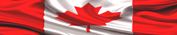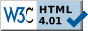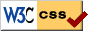HOME PAGE --------------- HELP+ » e-Design Notes --- » Switches, R, F » Resistors » Capacitors » Inductors » Diodes » Transistors --- » Potential Diff » Op Amps » Analog Filters --- » Boolean Algebra » Logic Gates » Flip-Flops » Logic ICs --- » Auto Injectors ---------------# ELECTRONIC DESIGN NOTES - POTENTIAL DIFFERENCE, CURRENT, IMPEDANCECommonly, people use the term "voltage" to name Electrical Potential Difference. Things are this way. Our Atomic Universe is composed of atoms, and we know about 118 of them. Each atom has a nucleus with positively charged particles named "protons". Around the nucleus, at a certain distance, there are tiny electrons spinning continuously, very fast, and they hold the mobile negative charges. The number of electrons varies from 1 to 118, plus or minus a few more. Important to remember is, the electrons are grouped in "electronic orbitals" around the nucleus.

In normal conditions, the electrons live happily within their electronic orbitals, and the atoms are balanced electrically--well, more or less. In particular conditions, however, the electrons on the last orbital may leave their rightful place, and in that case the atom becomes electrically charged, as follows:

1. if the electrons leave the last orbital, the atom becomes a "positively charged ion";
2. if external electrons come on the last orbital, the atom becomes a "negatively charged ion".

Because they can move form one atom to another, the electrons on the last orbital, in metals, are named "free electrons". Only free electrons can leave the atom, in normal conditions.

Electricity is a generic term used to name various effects produced by electrons. Historically, electricity is used on a large scale for about 100 years. However, electricity was known since old times.

The name of "electron" and the idea that it is a mobile electrical charge was first defined by Thales of Miletus (624-546 BC) during the Hellenic Civilization. However, there are some proofs that electricity was used 5000 years ago during the Egyptian Civilization, and even 7000 years ago during the Sumerian one. In order to present electricity in this page, the following (rudimentary) structure was employed:1. Electrostatics
2. Electric Potential Difference
3. Insulators and Conductors
4. DC current
5. AC current
6. Impedance
7. Kirchhoff's Laws

NOTE

The basic notions highlighted in this page are related to a few electronic design topics presented in the first part, Hardware Design, of
LEARN HARDWARE FIRMWARE AND SOFTWARE DESIGN.1. ELECTROSTATICS

Mr. Thales of Miletus did the following experience. He took an amber baguette, then he rubbed it vigorously with a piece of cloth. His baguette became charged with "electrostatic voltage". Next, he noticed the amber baguette was able to attract small pieces of cloth, and paper. That experience, plus many similar ones, helped him define the electron.

Later, his experience was repeated in laboratory conditions. The baguette was made of glass, at that time, and it was charged electrically by rubbing it with a piece of fur. The baguette was brought in touch with the sphere A, made of metal and isolated from ground via a wooden support. Next, the sphere A became charged positively as it is presented in Fig 1.

If a second sphere, B, was brought close to sphere A, it was noticed that sphere B also becomes electrically charged via "electrostatic induction" although overall on its surface it is still neutral electrically. The distribution of the electrical charges on sphere B surface is illustrated in Fig 1.

Fig1: Electrostatic InductionElectrostatic electricity
produces an "electrostatic field", very much similar to the magnetic one. Further, any body in proximity to an electrostatic field becomes electrically polarized.

A French scientist, Charles Augustine de Coulomb (1736-1785), has discovered that:

1. two isolated bodies charged with the same electric polarity repel each other;
2. two isolated bodies charged with different electric polarity attract each other.

The attraction or repulsion forces are caused by the electrostatic field, and they are sufficiently strong in close proximity to the charged bodies. Consequently, Mr. Coulomb has stated a Law that bears his name:

Coulomb's Law:    F  = k * Q1 * Q2 / r[N]

F is the attraction/repulsion force in Newtons
Q1 and Q2 are the values of the two electrical charges in Coulombs
r2 is the square distance between the two charged bodies in meters
k = 8.988*109 [N*m/C2] is a proportionality constant; the constant k was calculated as:
k = 1 / (4 * PI * ε)
PI = 3.141592 is the PI number
ε = 8.854 * 10-12 in [C2/(N*m2)] is "electric permittivity" in vacuum

Definition
One coulomb represents the electrical charge capable of repelling a similar electrical charge positioned one meter away, in vacuum, with a force of 8.988*109 [N]

Another great scientist, Mr. Michael Faraday (1791-1867), has made precise laboratory measurements, and he defined Electric Field Intensity.

Electric Field Intensity:   E  =  F / Q  [N/C]

The enigmatic electric field lines have a radial distribution around the electrically charged body. On the surface of an imaginary sphere, positioned at a certain distance from the electrically charged body, the intensity of the Electric Field is constant. By substituting in the above formula the force F with its expression calculated in Coulomb's Law, Electric Field Intensity becomes:

Electric Field Intensity for one electrically charged point (body):
E = (1 / 4*PI*ε) * (Q / r2)

A German scientist, Mr. Carl Friedrich Gauss (1777-1855), has calculated the number of electric lines passing through a closed surface (a sphere) enclosing an electric charge Q.

Gauss' Law:  N = Q / ε

N is the number of electric lines of force; it is independent of the radius
ε is electrical permittivity in vacuum2. ELECTRICAL POTENTIAL DIFFERENCE

Definition
Electrical potential difference between two electrically charged points A and B is the work needed to move charge "Q" from A to B

Electric Potential Difference:    ΔV  = VA - VB = W / Q

Definition
Electric potential difference between two points positioned 1 meter away is one volt if it requires one Joule of external work to move a charge of one Coulomb from one point to the other.

Volt definition:   1 [V] = 1 [J] / 1 [C]

Because W = F*d and F = Q*E we have W = Q*E*d and ΔV [N*m/C] = E * d

W is the work needed to move electrical charge q for distance d

NOTE
Electrical potential difference, also known as "voltage", is a relation/state between two electrically charged points; for example, the poles of a battery car. Technically, the two poles are "floating", unless we connect one pole to car's body. However, even in that case, the two poles are still floating when they are related to Earth's potential (true ground), because the body of the car is isolated by tires (rubber). Floating voltages need to be avoided, because they could reach very high voltages when related to Earth's (true) ground.3. INSULATORS AND CONDUCTORS

We know 118 atomic elements, and about two hundred thousand substances, natural and synthetic--a substance is built out of molecules which in turn are composed of atoms (or elements). All elements and substances may be electrically polarized, but not all of them allow the conduction of electrical current. Generally, metals are known to be good electrical conductors.

Electrical current conduction is a complex phenomenon. Suppose we connect the poles of a battery via a metallic wire. Electrical current starts "flowing" through that conductor almost instantly, because it "moves" at light-speed (2.998*108 [m/s]). The way this "current flow" happens is characterized by the following considerations:

1. in order to have current flow, we need first of all an Electrical Potential Difference;
2. as soon as we do have Electrical Potential Difference, on the surface of the conductor ONLY free electrons start jumping to the adjacent atoms;
3. the "flow of current" is in fact billions of small jumps executed by billions of free electrons from one atom to the next one, ONLY. That means, it is highly unlikely one electron will jump through the entire length of the conductor;
4. the direction of the electrons movement (the jumps) is from the negative pole (-) to the positive one (+). However, people/scientists have decided the positive sense of the current is from (+) to (-);
5. the intensity of the electrical current is controlled only by the resistance of the conductor.

NOTE
The first condition above may be replaced by: "in order to have a current flow, we need to excite the atoms using:
1. Electrical Potential Difference;
2. light;
3. temperature;
4. moving magnetic or electromagnetic fields;
5. moving electrostatic fields;
7. many more."

Definition
The resistance of a conductor (R) is directly proportional to its resistivity (ρ) and the length of the conductor (L), and inversely proportional to the cross-sectional area (A) of that conductor. Resistance is measured in ohms.

Resistance:  R = ρ * L / A  [Ω]

"ρ" in the above formula should be read as "RO": it is a letter of the Latin alphabet used to mark resistivity, a constant particular to each material (both to conductors and insulators)
A is the cross-sectional area
Ω is the symbol for ohm

Definition
A conductor is a material (substance, pure element, alloy) which allows the electric current to flow. For metallic conductors resistivity has very low values.

Definition
An insulator (or non conductor) is a material (substance, pure element, alloy) which does not allow the electric current to flow, in normal conditions. That means, its resistivity is very high.4. DC CURRENT

Electrostatic electricity doesn't help us much because:
1. the electrostatic voltages are too high;
2. it ends up in an instance/flash once shorted by conductors.

For practical applications, we need continuous electric current having (relatively) low voltages. The most known source of DC (Direct Current) is a chemical battery, marked with E, and you can see its schematic representation in Fig2.

Ohm's Law:  R = U / I   [Ω]

In Fig 2 Ohm's Law is calculated as: 1000 [Ω] = 12[V] / 0.012[A]Fig 2: An elementary DC circuit5. AC CURRENT

The AC (Alternative Current) is supplied by the power utility grid, and it alternates with a frequency of:
1. 50 Hz in Europe (and most of the World)
2. 60 Hz in N America (and in a few more places)

What everybody should know is, both 50 Hz and 60 Hz are very dangerous for the human body. For example, if you touch a 110 V 60 Hz live wire you could die; if the frequency is 120 Hz the danger is way lower; at 300 Hz frequency things are far safer.

Transformers and electrical motors built for 50 Hz work (relatively) well and safe at 60 Hz; if they are built for 60 Hz, however, they may not work well at 50 Hz--they generate excessive heat, slower speeds, hum/noise, etc. Due to the alternating frequency (f) the instantaneous voltage and current (at time t) in the AC circuit picture in Fig 3 is:

V(t) = Vmax * sin (2 * PI * f * t)
I(t)  = Imax  * sin (2 * PI * f * t)

Please note in Fig3 that one pole is grounded, although that is not mandatory. However, ungrounded circuits are floating, and they may reach very high voltages; by grounding them "we pull the voltage down to the ground reference potential". All AC circuits are grounded. In Fig 3 the "live" wire is violet in color, and the "marked" (or polarized, or grounded) one is blue.

Fig 3: An elementary AC circuitNote in Fig 3 that we have a load R of 10 kilo ohms: it is a resistive load. That is the reason  the AC current sine wave in Fig 4 follows the AC voltage exactly.

Fig 4: Voltage and Current in AC resistive circuitsPlease take a look at the above two pictures; note the following:
1. if the load is inductive, the AC current will "lead" the voltage sine wave by PI/4
2. if the load is capacitive, the AC current will "lag" the voltage sine wave by PI/4

The following formulas are employed to define the AC flow:
Effective voltage is:   Veff = 0.707 Vmax
Effective current is:    Ieff = 0.707 Imax
Maximum voltage is:   Vmax = 1.414 Veff
Maximum current is:    Imax  = 1.414 Ieff
The period T of the AC current/voltage sine wave is:   T = 1 / f6. IMPEDANCE

In AC circuits the Ohm's Law needs to be written as:

Ohm's Law in AC circuits:  Z = U  / I   [Ω]

Z in the above formula is the "impedance" in ohms of the circuit pictured in Fig 5, and it is calculated with:

Impedance:   Z = √[R2 + (XL - XC)2]

The impedance formula has two terms; they are:

Inductive Reactance:   XL = 2 * PI * f * L
Capacitive Reactance:  XC = 1 / (2 * PI * f * C)

Fig 5: Impedance in AC circuitsATTENTION

In most DC circuits we work with pulses having two DC states:
1. False, for a DC voltage of 0 V
2. True, for a DC voltage of +5 V

However, each time a pulse changes its state from True to False, and back, that DC circuit ceases to be a DC one: it becomes an AC/pulsing circuit, therefore all its elements need to be (re-)calculated accordingly!

NOTE
In AC circuits (including most DC ones--see the above ATTENTION) when the frequency becomes very high, Inductive Reactance increases a lot, and the inductor behaves like an interrupted circuit--similar to a capacitor.

NOTE

In AC circuits (including most DC ones--see the above ATTENTION) when the frequency becomes very high, Capacitive Reactance also increases, therefore capacitors behave like resistors having very low values. Due to that aspect, capacitors are said they have a "leakage/parasitic current".7. KIRCHOFF'S LAWS FOR ELECTRICAL CIRCUITS

Kirchoff's Laws
for electrical circuits are sufficient to calculate all currents and voltages in any section of any circuit. They are:

Definition
The algebraic sum of the currents at a junction is zero.

Kirchoff's Law for electrical nodes:
Σ I = 0

NOTE
In the above formula, the currents entering the junction/node have the (+) sign; if they leave that junction the currents take the (-) sign. According to Kirchoff's Law, the currents of a junction/node are summed algebraically.

Examples:
Node A in Fig 6:
9.4[mA] - 6.4[mA] - 3[mA] = 0

Node E:
2.4[mA] + 4[mA] - 6.4[mA] = 0

Definition
In any closed loop of an electric circuit the algebraic sum of the generated voltages is equal to the algebraic sum of the potential drops (differences) existing on that loop.

Kirchoff's Law for electrical loops:
Σ E = Σ( I * R)

Examples:
Loop XAFY in Fig 6:
12[V] = 0.003[A]*4000[ohm]

Loop ABEF:
0[V] = 0.0024[A]*5000[ohm] - 0.003[A]*4000[ohm]

Please use Fig 6 to test the two Laws. The generated voltage E is 12V, and Potential Drops are developed on each resistor. Examples of closed loops are:
# XAFY; XABEFY; ABEF; BCDE; etc

NOTE
In the second Law, the current keeps its sign, and potential drops are summed algebraically, considering the CW (clockwise) direction to be the positive one.

Fig 6: Kirchhoff's LawsTake control of the extraordinary IT Revolution with Learn Hardware Firmware and Software Design--the easy way!» LINK TO "LOGICALLY STRUCTURED ENGLISH GRAMMAR"Page last updated on: July 25, 2018Site pages valid according to W3CStylesheets pages valid according to W3C